Early Measurements of the Band Effective Mass in Silicon Using Cyclotron Resonance

S. L. Hellstrom May 29, 2007

(Submitted as coursework for Applied Physics 273, Stanford University, Spring 2007)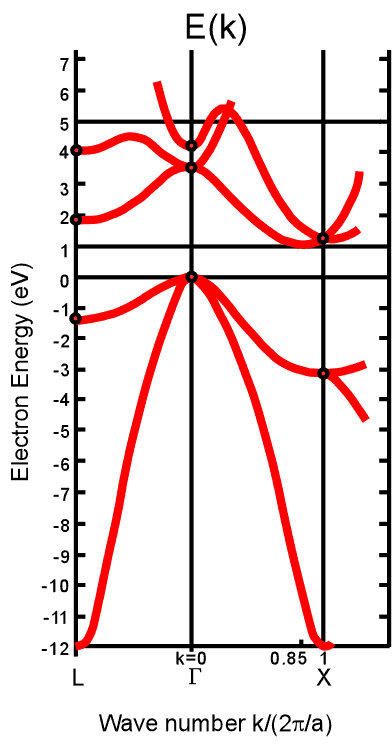Figure 1: Band structure of silicon. 

Concept of Effective Mass

The dispersion relation of free electrons is: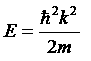In a crystalline solid, the relationship between energy and momentum, given by the band structure or E-k diagram of the crystal, is considerably more complex than that of the free electron dispersion relation. Arguably the most important example is silicon, whose band structure is shown in Figure 1.  However for silicon and other semiconductors one may take advantage of the fact that the majority of charge carriers are located (in k-space) near the conduction band minima or valence band maxima, and thus the dispersion relation may be approximated as quadratic, i.e.,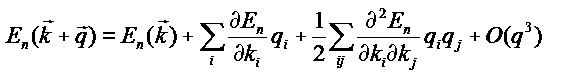q is a small displacement from the band minimum or maximum; i and j are spatial coordinates and n indicates the energy level of the carrier.  By direct analogy to the free electron case, the coefficient of the q2 term in the expansion is set to equal (h/2π)2/ 2m*, where the effective mass tensor is defined for a general E(k):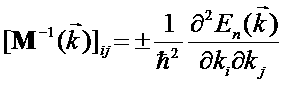The sign is (-) or (+) according to whether k is near a band maximum, for holes, or minimum, for electrons, to render the sign of the effective mass positive. Each component of M refers to an effective mass in a particular direction and at a particular k. In practicality then, near the bandgap, to second order the impact of the semiconductor band structure is simply a change of the apparent mass of the charge carrier in the medium.

The concept of effective mass is useful in two principal ways. Firstly, band structures for most solids cannot be obtained analytically. Rather, scientists and engineers use the effective mass to develop simple models for charge carrier behavior in semiconductor devices, that avoid the detailed physics of the E-k diagram yet nevertheless reproduce the basic behavior of the system in question. Secondly, while the effective mass tensor may be written in terms of a perturbation theory expansion of the general system Hamiltonian  it is also readily determinable experimentally (for a semiconductor at least), and therefore measurement of the effective mass in a material is a useful probe of its E-k structure near the band gap, and a useful corroboration of the band theory of solids. 

The situation is made a bit more complicated because the minima and maxima of silicon are anisotropic, and further, as can be inferred from Figure 1, there are six equivalent identical conduction band minima at the band edge. There are also two different valence band maxima at k=0 and equal E. The effective mass actually used in an engineering calculation therefore combines elements of the mass tensor in different ways, depending on the calculation.  For example, the conductivity of a material is inversely proportional to the effective mass. So, the conductivity due to distinct multiple band maxima or minima is proportional to the sum of the inverse of the individual masses (as in the addition of parallel resistors) multiplied by the density of carriers in each band, to reflect the amount each maximum or minimum adds to the overall conductivity. Further if the extrema are anisotropic, one must sum over the effective masses in the different minima along the equivalent directions. Determination of the effective mass to use in a calculation, from the components of the effective mass tensor, therefore requires some consideration.

Theory of Cyclotron Resonance

One of the most straightforward methods of measuring effective mass in a semiconductor is to study the behavior of the carriers in the presence of a magnetic field. The theory of this behavior may be developed semiclassically [2,5] or quantum mechanically. We are concerned with the energy levels in the presence of a uniform static field Ξ.

In many cases the semiclassical analysis is sufficient. Each carrier is assumed to independently obey the equation of motion: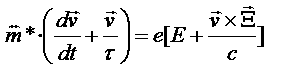τ is the scattering lifetime, assumed a constant. Then J(E)=nEv=σE where J is the current density, n is the carrier density and σ is the complex conductivity tensor. Under the assumptions of a cubic material with the magnetic field in the z-direction, and for an incident wave linearly polarized in the x-direction, the power absorbed may be readily computed as: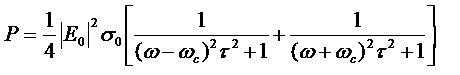σ0=Ne2τ/m* is the static conductivity, and ωc=|eB|/m*. So there will be a resonance whenever ω=ωc and ωcτ>1.

From a quantum mechanical perspective, the general Hamiltonian of an electron in the presence of a magnetic field is: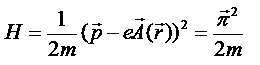where π is an operator often called "kinematic momentum"  that incorporates the applied magnetic field and satisfies canonical commutation relations. Therefore they may be written in terms of ladder operators and the Hamiltonian recast in the form of a simple harmonic oscillator with eigenfrequencies ωc=|eB|/m*. Each eigenvalue is associated with a highly degenerate set of spiral orbits known collectively as a Landau level. In the electric dipole approximation, when shining polarized light on the sample the Hamiltonian becomes H=H0+H' where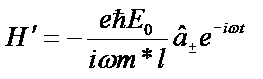So the perturbation connects state n with state n+1 or n-1 and a sharp absorption occurs at ω=ωc.

Now ωc is a parameter dependent only on fundamental constants, an applied magnetic field, and the mass of the electron. So in either case by applying a magnetic field to a semiconductor, and by measuring transmittance of a beam of long-wavelength light shining on the semiconductor, one may observe via strong absorption bands where the cyclotron frequencies are, and hence almost directly the mass of the spiraling electron.

Experimental Determination of Effective Masses in Silicon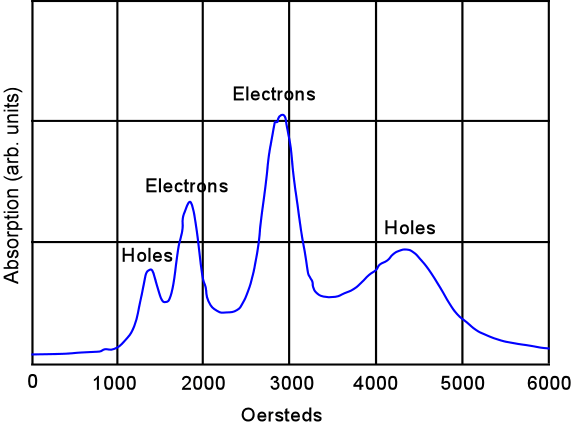Figure 2: Sample absorbance vs. magnetic field data for silicon. The static magnetic field orientation is in a (110) plane 30 degrees from a  axis. 

In 1954, Dresselhaus, Kip and Kittel reported the first measurements of effective masses in silicon, using the basic cyclotron resonance technique described in the previous section; that is, by applying a magnetic field and long-wavelength (microwave) radiation to a silicon sample and looking for peaks in radiation absorption such as those shown in Figure 2. To get the light through the sample it was required that the sample be thin and have a sufficiently low carrier density; increasingly conductive samples suffered depolarization and attenuation. Samples used were therefore 3mm x 0.5mm, made by cutting and etching from larger single crystals. Samples were mounted on a rotating base to enable study as a function of crystal orientation.

To improve the experimental signal to noise ratio, energy absorption under cyclotron resonance was determined by measuring the change in Q of a microwave cavity in which the sample has been placed. Some of the power reflected back from the test cavity reached a crystal detector; a change in the loaded Q of the cavity indicated cyclotron absorption by a change in the reflected power. The input was modulated so there was an ac signal at the crystal which then passed through an amplifier and lock-in detector. The magnetic field was then varied slowly to determine power absorption versus magnetic field.

As mentioned previously, since spectral line width is determined by the collision relaxation time τ, ωcτ must be greater than 1 to observe distinctive resonances. This is equivalent to saying that the mean free path of the carrier must be large enough to get approximately 1/(2π) of the way around a circle before scattering against lattice vibrations, impurity atoms and the like. In order to get a sufficiently long (about 10-13-10-15 seconds) relaxation time, low temperature and high purity samples are required. For this reason experimental samples were generally immersed in liquid He for measurement around 4K.

Unfortunately, at the low temperatures required to sufficiently lengthen the mean free path, the equilibrium charge carrier density in Si is too small to allow observation of resonance absorption, and the incident microwaves cannot excite sufficient carriers as they may for Ge. Free carriers were produced either by application of a modulated light beam or by application of an AC voltage. The light method generated electron-hole pairs, however, so both electron and hole resonances were observed in these cases. The preferred method for silicon was thus to use an applied AC voltage to generate the carriers, in which case the sample was placed just outside a small hole in the cavity to alleviate the difficulties of getting wires into the cavity itself.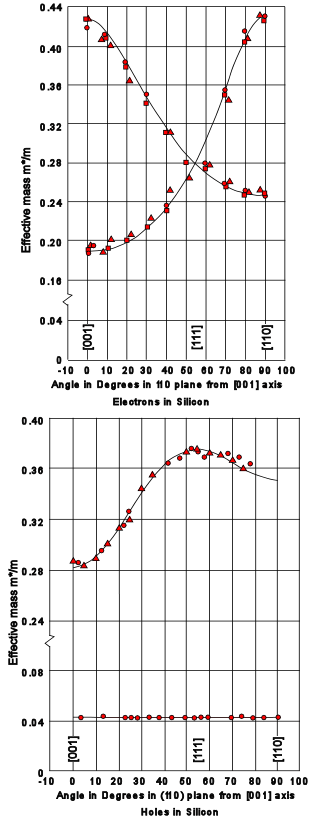Figure 3: Effective masses of Silicon measured and compared with theory. 

The end results of these experiments are shown in Figure 3. How does one connect theory with experiment, e.g., determine the effective masses from the observed cyclotron resonance behavior? Shockley  gives the solution of the classical equations of motion for a general ellipsoidal energy surface, which is valid in the case of conduction band electrons in silicon. The effective-mass Hamiltonian is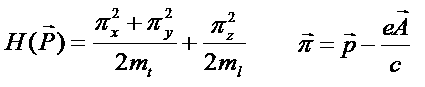Setting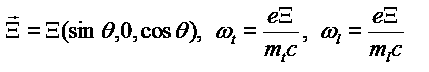Then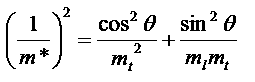where m* in this case denotes the effective mass determining the cyclotron frequency when the static magnetic field makes an angle θ with the longitudinal axis of the energy surface. By symmetry there are six equivalent minima in the conduction band of silicon, at at k = (x,0,0), (-x,0,0), (0,x,0), (0,-x,0), (0,0,x), and (0,0,-x) with x=5nm-1.  Fitting absorbance data to the equation above gives m*e,l=0.97me and m*e,t=0.19me.

The behavior of the valence band holes is more complex, because of the threefold orbital degeneracy (not considering spin-orbit coupling) of the valence band at k=0. The energy surfaces are warped spheres, and consequently there is no longer a single frequency for a given magnetic field orientation, rather a distribution. Shockley  also first discussed this problem, for the full degeneracy. In silicon, spin-orbit coupling splits off one of the maxima so it is no longer quite degenerate. The theory is too detailed to be presented here in full extent; it was developed in  itself, using perturbation theory expanding in pseudo-Bloch functions of the form u(k,r)eikr. After generalization to include spin-orbit splitting, the roots of the total perturbation Hamiltonian matrix are of the form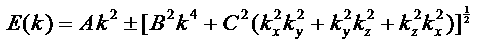If it is assumed that a semiclassical treatment is valid hereon in, one may assume a general effective mass Hamiltonian H(P) where P=hk/2π. The magnetic field in a classical limit does not change the energy of a particle moving on an energy surface, nor does it change the projection of P along the magnetic field direction. From the equations of motion,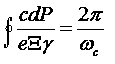where γ is the magnitude of the projection of the group velocity on a plane perpendicular to the magnetic field. A similar integral expression for m* may be determined by defining ωc in terms of m* although it is very difficult to solve this equation in the general case. For the magnetic field parallel to the (110) direction m* may be expanded in terms of A, B, and C to give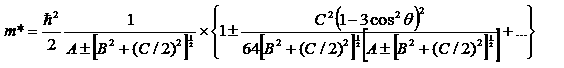with an error not greater than about 1%. θ is the angle the magnetic field makes with the  direction.  After measuring the cyclotron resonance frequencies then, the experimental effective masses may be fitted to the above equation and A, B, and C determined. In Si it was found A=-4.1 (h/2π)2/ 2m, |B|=1.6 (h/2π)2/ 2m), and |C|=3.3 (h/2π)2/ 2m. It is clear from Figure 3 that there is a "heavy hole" and a "light hole" solution, with a light hole mass of 0.16me and a heavy hole mass of 0.46me, in the (110) plane. The split off band, not shown in the figure, has a mass of approximately 0.29me.

© 2007 S.L. Hellstrom. The author grants permission to copy, distribute and display this work in unaltered form, with attribution to the author, for noncommercial purposes only. All other rights, including commercial rights, are reserved to the author.

References

 C.-T. Sah, Fundamentals of Solid State Electronics, (World Sci., 1991).

 N.W. Ashcroft, N.D. Mermin, Solid State Physics (Thompson Learning, 1976).

 J. M. Luttinger, W. Kohn, "Motion of Electrons and Holes in Perturbed Periodic Fields," Phys. Rev. 97, 869 (1995).

 B. V. Zeghbroeck, Principles of Semiconductor Devices, http://ece-www.colorado.edu/~bart/book/welcome.htm.

 G. Dresselhaus, A.F. Kip and C. Kittel, "Cyclotron Resonance of Electrons and Holes in Silicon and Germanium Crystals," Phys. Rev. 98, 368 (1955).

 J. Kono "Cyclotron Resonance," Methods in Materials Research, ed. E. N. Kaufmann et al. (Wiley, 1999).

 J.J. Sakurai, Modern Quantum Mechanics, ed. S.F. Tuan (Addison-Wesley, 1994).

 W. Shockley, "Cyclotron Resonances, Magnetoresistance, and Brillouin Zones in Semiconductors," Phys. Rev. 90, 491 (1953).

 W. Shockley, "Energy Band Structures in Semiconductors," Phys. Rev. 78, 173 (1950).